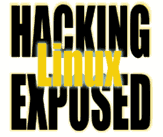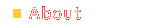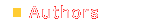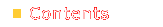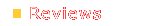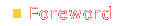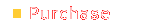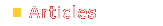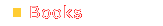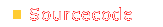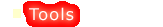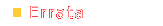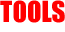```#!/usr/bin/perl # # Quick dirty dvorak to/from querty script # # This is *NOT* the script I used for the book. # (You'd know why if you read it.) # # This is also an execellent example of how not to # write readable code. # # Writing these comments took longer than writing # the code itself. # # Bri Hatch # Released under the GPL use strict; use Getopt::Long; # Our big lookup table my @mapping = qw( = ] ' q , w w , . e v . z / ; z s ; [ - - ' / [ ] = a a o s j c q x e d k v u f y t p r b n x b d h i g f y m m h j g u t k c i r o n l l p ); use warnings; # Here, due to warning in mapping above. # Make hashes from the array my(%tod,%toq); while ( @mapping ) { my(\$d,\$q) = splice(@mapping,0,2); \$toq{uc \$d} = uc \$q; \$tod{uc \$q} = uc \$d; \$toq{\$d} = \$q; \$tod{\$q} = \$d; } my(\$tod,\$toq); GetOptions( 'tod|d' => \\$tod, 'toq|q' => \\$toq ); die "Usage: \$0 {--todvorak | --toqwerty}\n" unless (\$toq or \$tod) and not (\$toq and \$tod); while (<>) { if ( \$toq ) { s/(.)/ defined \$toq{\$1}? "\$toq{\$1}":"\$1" /eg; print; } else { s/(.)/ defined \$tod{\$1}? "\$tod{\$1}":"\$1" /eg; print; } } ```